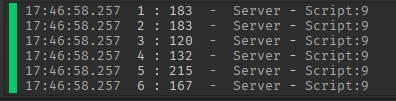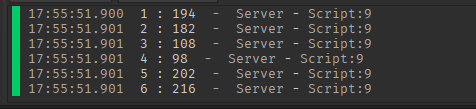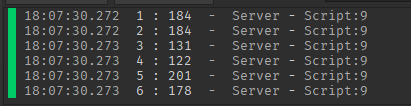# Dice script not working properly

so i made a dice script for my game and it works fine, but after running a few tests i noticed that for some reason 3 and 4 are rarer than the rest of the numbers but i have no idea why``````module.rollDice = function(dice)
local faces={
["Back"]=5,
["Bottom"]=6,
["Front"]=2,
["Left"]=4,
["Right"]=3,
["Top"]=1
}

local function getsurface(surfacenormal)
local normal = dice.PrimaryPart.CFrame:VectorToObjectSpace(surfacenormal)
local nx,ny,nz = math.round(normal.X),math.round(normal.Y),math.round(normal.Z)
if nx ~= 0 and (ny == 0 and nz == 0) then
if nx > 0 then
return("Front")
else
return("Back")
end
elseif ny ~= 0 and (nx == 0 and nz == 0) then
if ny > 0 then
return("Top")
else
return("Bottom")
end
elseif nz ~= 0 and (nx == 0 and ny == 0) then
if nz > 0 then
return("Left")
else
return("Right")
end
end
end
Tween1:Play()
Tween1.Completed:Wait()
Tween2:Play()
Tween2.Completed:Wait()
Tween3:Play()
Tween3.Completed:Wait()
local params = RaycastParams.new()
params.FilterType = Enum.RaycastFilterType.Whitelist
params.FilterDescendantsInstances = {dice.PrimaryPart}
local origin = dice.PrimaryPart.Position+Vector3.new(0,dice.PrimaryPart.Size.Y,0)
local results = workspace:Raycast(origin,dice.PrimaryPart.Position-origin,params)
local surface = getsurface(results.Normal)
return faces[surface]
end
``````
1 Like

Does the math.random seed change at all? Also consider running a lot more tests than that since it might just be naturally biased against 3 and 4 for the first 1000 rolls or something

1 Like

Consider changing the `math.random` seed everytime the dice rolls to `os.time()`, sometimes `math.random` can be a bit biased if the seed remains constant:

``````--on dice roll
math.randomseed(os.time())
``````
1 Like

`os.time()` isn’t exactly reliable since it means it will only change the seed every second so you’re going to get the same exact results if you use math.random multiple times in that one second interval

I would use something like this

``````--seed will change every microsecond with this method
--you might have to truncate the number so it doesn't overflow and break
math.randomseed(math.floor(os.clock()*1e6))
``````

http://lua-users.org/wiki/MathLibraryTutorial

2 Likes

i dont really get it, is this what i am supposed to do?

``````local seed = math.randomseed(math.floor(os.clock()*1e6))
Tween1:Play()
Tween1.Completed:Wait()
Tween2:Play()
Tween2.Completed:Wait()
Tween3:Play()
Tween3.Completed:Wait()
``````

`math.randomseed` is a void function, you simply just run it and it will change the sequence of `math.random`
You’re supposed to change the seed right before calling `math.random`, every time

Here’s an example of how to use it

``````for _ = 1, 100 do
math.randomseed(math.floor(os.clock()*1000000))
print(math.random(1, 100))
end
``````

now the dice is only rotating in 1 direction

``````math.randomseed(math.floor(os.clock()*1e6))
Tween1:Play()
Tween1.Completed:Wait()
Tween2:Play()
Tween2.Completed:Wait()
``````local intClock = tostring(os.clock()*1e6)